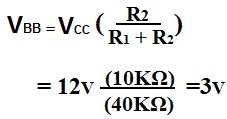﻿ How to Calculate VBB of a Transistor# How to Calculate VBB of a Transistor

VBB, the base voltage of a bipolar junction transistor, or in other words, the voltage that falls across the base of the transistor, is crucial to calculations such as when calculating the base current, IB or the quiescent emitter current, IEQ.

VBB is calculated by the formula below:ExampleRelated Resources